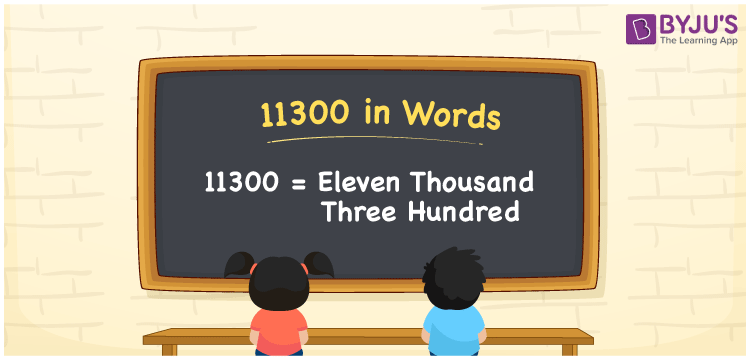# 11300 in Words

11300 in words is written as Eleven thousand three hundred. In both the International System of Numerals and the Indian System of Numerals, 11300 in words is written as Eleven thousand three hundred. The number 11300 is a Cardinal Number as it represents some quantity. For example, “he has 11300 rupees in his bank account”.

 11300 in words Eleven thousand three hundred Eleven thousand three hundred in numerals 11300

## 11300 in English Words

11300 in English words is read as “Eleven thousand three hundred.”## How to Write 11300 in Words?

To write 11300 in words, we shall use the place value chart. In the place value chart, put 1 in the ten thousands, 1 in the thousands, 3 in the hundreds, and 0 in the tens and the ones, respectively. Let us make a place value chart to write the number 11300 in words.

 Ten Thousands Thousands Hundreds Tens Ones 1 1 3 0 0

Thus, we can write the expanded form as

1 × Ten Thousand + 1 × Thousand + 3 × Hundred + 0 × Ten + 0 × One

= 1 × 10000 + 1 × 1000 + 3 × 100 + 0 × 10 + 0 × 1

= 10000 + 1000 + 300 + 0 + 0

= 11300

= Eleven thousand three hundred.

11300 is a natural number between 11299 and 11301.

11300 in words – Eleven thousand three hundred

• Is 11300 an odd number? – No
• Is 11300 an even number? – Yes
• Is 11300 a perfect square number? – No
• Is 11300 a perfect cube number? – No
• Is 11300 a prime number? – No
• Is 11300 a composite number? – Yes

## Frequently Asked Questions on 11300 in Words

Q1

### How to write 11300 in words?

11300 in words can be written as Eleven thousand three hundred.
Q2

### How to write 11300 in words in the International and Indian System of Numerals?

In both, the system of numerals, 11300 in words, is written as Eleven thousand three hundred.
Q3

### How to write 11300 in a place value chart?

In the place value chart, write 1 in the ten thousands, 1 in the thousands, 3 in the hundreds, and 0 in the tens and the ones, respectively.
Test your Knowledge on 11300 in Words